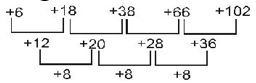# Number Series Questions

Q:

What comes next in the series...

1A2, 3C4, 7G8, ___

 A) 15O16 B) 16P17 C) 14O15 D) 13P14

Explanation:

The first digit is formed by adding the numbers which are the results of powers of 2 increased by one and second letter is the corresponding alphabet to that number...last number is the next number to the first one...therefore

15=7+8 (i.e. 2^3)

O is corresponding alphabet to 15

and 16 comes after 15

40 37975
Q:

What will come in place of question mark(?) in the following number series ?

6859       5832     ?      4096     3375

 A) 4589 B) 4913 C) 5013 D) 5169

Explanation:

The given series follows a pattern that,

19 x 19 x 19 = 6859

18 x 18 x 18 = 5832

17 x 17 x 17 = 4913

16 x 16 x 16 = 4096

15 x 15 x 15 = 3375

So, the missing number in the given series = 4913

87 35700
Q:

Complete the number series given below ?

13        19       37      ?         141        243

 A) 58 B) 67 C) 75 D) 96

Explanation:

The given series follows the pattern thatSo the missing number is  37 + 38 = 75

75 + 66 = 141

....

84 34942
Q:

Find the next number in the given number series?

6, 6, 12, 36, 144, 720, ?

 A) 4320 B) 3547 C) 2154 D) 1765

Explanation:

The given series is 6, 6, 12, 36, 144, 720, ?

6

6 x 1 = 6

6 x 2 = 12

12 x 3 = 36

36 x 4 = 144

144 x 5 = 720

720 x 6 = 4320

Hence, the next number in the given number series is 4320.

27 34147
Q:

Choose the missing terms out of the given alternatives Z, X, S, I, R, R, ?, ?

 A) J, I B) K, M C) G, I D) J, K

Explanation:

Z - 2 = X
X - 5 = S
S - 10 = I
I - 17 = R
R - 26 = R
R - 37 = G
G - 50 = I
The series 2, 5, 10, 17, 26, 37, 50 is obtained by the pattern +3, +5, +7, +9, . . . .

241 33291
Q:

Find the wrong number in the following series?

1500, 1475, 1448, 1419, 1389, 1355, 1320

 A) 1475 B) 1419 C) 1389 D) 1355

Explanation:

Take a look at the below sereies :

1500 - 25 = 1475

1475 - 27 = 1448

1448 - 29 = 1419

1419 - 31 = 1388

1388 - 33 = 1355

1355 - 35 = 1320

It clearly shows 1389 is Wrong Number

28 33069
Q:

Look at the series :

3, 7, 24, 11, __, 19, 19,... What number should fill the blank ?

 A) 15 B) 13 C) 21 D) 16

Explanation:

Two series alternate here, with every third number following a different pattern.
In the main series, 4 is added to each number to arrive the next.
In alternate series, 5 is subtracted from each number t arrive the next number.
Here in the main series
3 + 4 = 7
7 + 4 = 11
11 + 4 = 15
15 + 4 = 19

In alternate series
24 - 5 = 19
19 - 5 = 14...

36 31957
Q:

Find the next number in the given number series

1, 3, 10, 41, 206, ?

 A) 1237 B) 897 C) 987 D) 1007

Explanation:

The given number series is 1, 3, 10, 41, 206, ?

It follows a pattern that,

1

1 x 2 + 1 = 3

3 x 3 + 1 = 10

10 x 4 + 1 = 41

41 x 5 + 1 = 206

206 x 6 + 1 = 1237

Hence, the next number in the given number series is 1237.